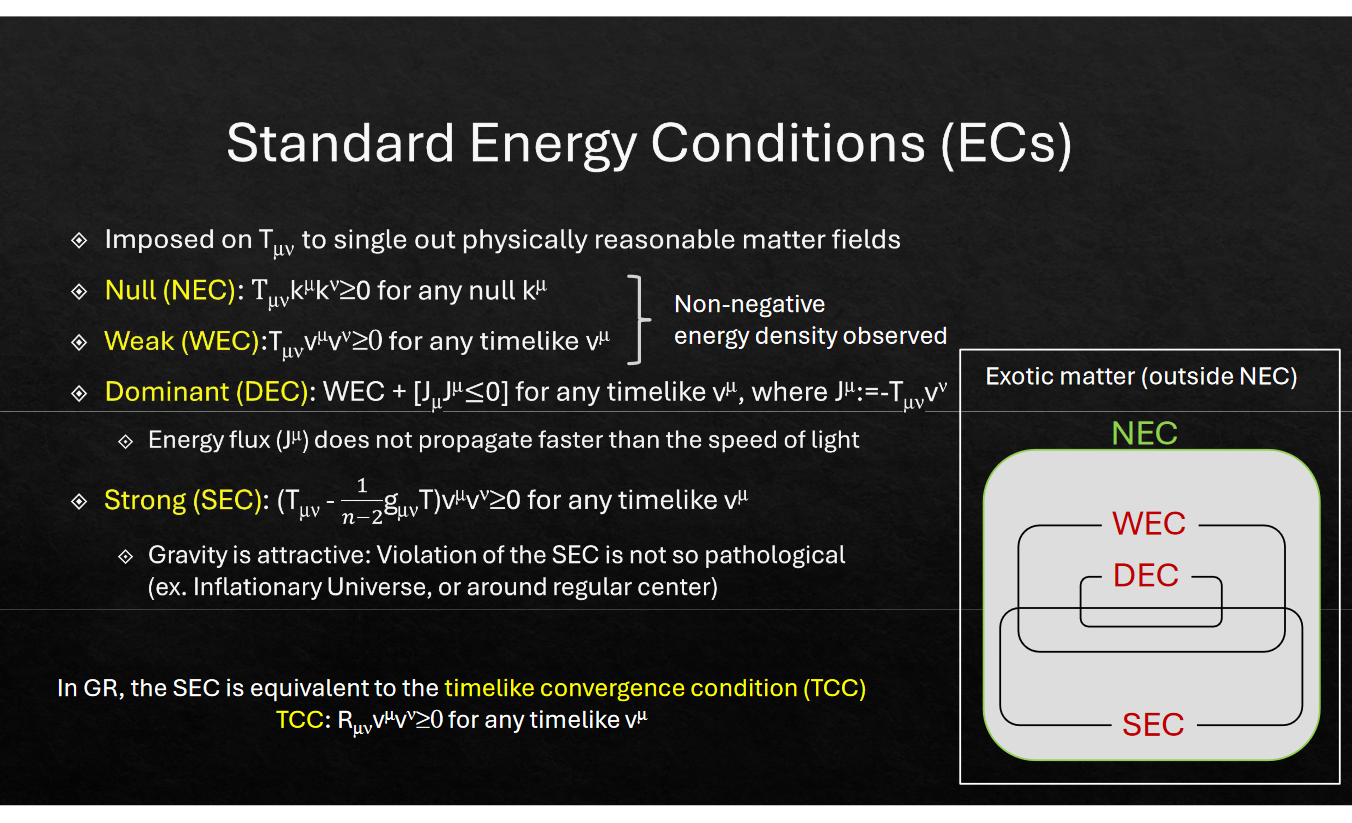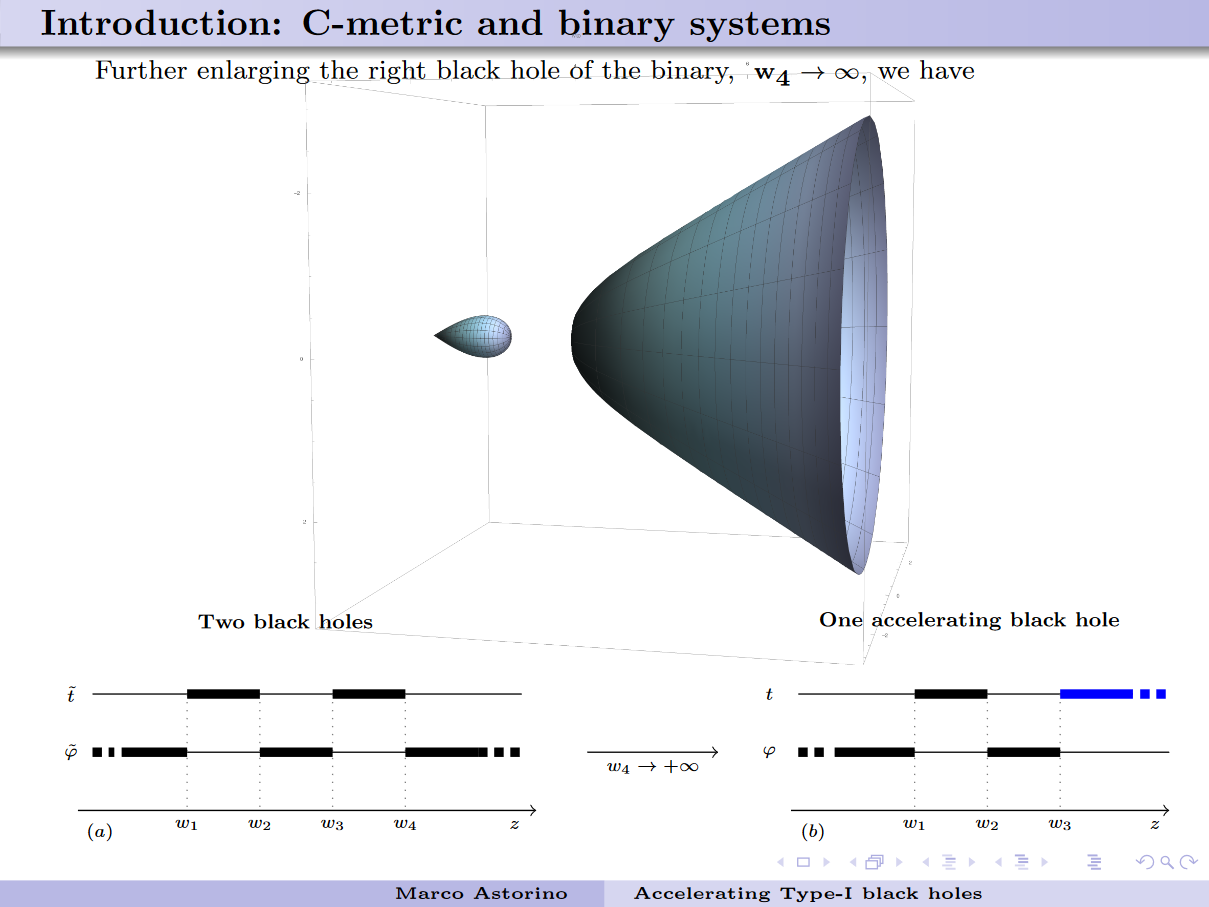# Relativistický seminářÚstavu teoretické fyziky

Seminář se koná v úterý ve 13:10 v posluchárně ÚTF MFF UK
v 10. patře katedrové budovy v Tróji, V Holešovičkách 2, Praha 8

## Příští semináře:

12. prosince 2023
Static metric with a ring source in toroidal coordinates
doc. Tomáš Ledvinka
ÚTF
(seminář v angličtině)

In 1975, in a short paper named "A toroidal solution of the vacuum Einstein field equations", Kip S. Thorne suggested that an external field of gravitating toroidal source requires introducing cuts and identifications in order to be expressed in Weyl coordinates with their attractively simple form of field equations. Since then only 10 papers seem to have this result in their references and only one actually uses Thorne's result. Among others, I will show how these cuts and identifications necessarily arise from the analytic properties of transformations leading to Weyl metric, what coordinates should be used to get smooth metric, and how one can find metric of this spacetime numerically.

19. prosince 2023
Measuring the temperature of quantum fields: Unruh and Hawking effects
Tales Rick Perche
Perimeter Institute for Theoretical Physics / University of Waterloo
(seminář v angličtině)

In this talk we will show that if the field is in a state that satisfies the KMS condition with inverse temperature beta with respect to a detector's local notion of time evolution, reasonable assumptions ensure that the probe thermalizes to the temperature 1/beta; in the limit of long interaction times. This is true regardless of the field operator that the detector couples to. Our method also imposes bounds on the size of the system with respect to its proper acceleration and spacetime curvature in order to accurately probe the KMS temperature of the field. We then comment on applications to the case of detectors probing the Unruh and Hawking temperatures.

## Minulé semináře:

26. září 2023
Love symmetry in higher-dimensional rotating black hole spacetimes
Dr. Finnian Gray
Gravitational Physics, University of Vienna
(seminář v angličtině)
We develop a method for constructing globally-defined Love symmetry generators in rotating black hole spacetimes of a general dimension. After revisiting the 4D Kerr and 5D Myers-Perry cases, the procedure is illustrated on generalized Lense-Thirring spacetimes which describe a wide variety of slowly rotating black hole metrics in any number of dimensions. Such spacetimes are known to admit an extended tower of Killing tensor and Killing vector symmetries and, as demonstrated in this paper, allow for separability of massive scalar equation in standard Myers-Perry-like coordinates.
3. října 2023
Entropy bounds for AdS black holes
Dr. Robie A. Hennigar
Institute for Cosmic Sciences, University of Barcelona
(seminář v angličtině)
Extended black hole thermodynamics concerns the study of pressure and volume terms in the first law. One of the early results in this field was the conjectured reverse isoperimetric inequality (RII), which bounds the black hole area in terms of the thermodynamic volume. In this talk, I will discuss recent work that generalizes the isoperimetric inequality generating new conjectured bounds on black hole entropy.
10. října 2023
The gravitational wave phase at 4.5PN order and the tails of memory
Dr. David Trestini
Laboratoire Univers et Théories (LUTH), Meudon, nyní CEICO, Fyzikální ústav AV ČR
(seminář v angličtině)

The main observable for the detection of gravitational waves in general relativity generated by compact binary systems is the phase of the wave. Within the post-Newtonian approximation, where the objects are assumed to be well-separated and to have small velocities, one can obtain analytical expressions for the phase as a series in $(v/c)^2$. Before this work, the phase (as well as the complete waveform) was known up to 3.5 post-Newtonian (PN) order. In this talk, I will discuss our recent computation of the flux and phase at 4.5PN order, as well as the $(\ell,m)=(2,2)$ mode at 4PN order. I will give a brief overview of the different steps leading to this results, then will discuss more in detail my work on the tails of memory. Finally, I will discuss what is needed to obtain all the $(\ell,m)$ modes entering the 4PN waveform, and how the tail-of-memory methodology will be central to it.

17. října 2023
Even photons break de Sitter symmetry
Dr. Dražen Glavan
CEICO, Institute of Physics of the Czech Academy of Sciences
(seminář v angličtině)

It is commonly held that the propagator for the massless vector field in de Sitter in the general covariant gauge has to respect all the symmetries of the maximally symmetric background. However, this is precluded by the Ward-Takahashi identity that has gone unnoticed thus far. I will present the recent construction of the photon propagator that satisfies all the conditions of a consistently quantized theory, and that maintains all de Sitter symmetries except spatial special conformal transformations. This propagator vanishes in the infrared, opposed to previously reported solutions that satisfied just the propagator equation of motion. Even though the de Sitter breaking pertains to the gauge sector, it is important when interactions are considered. I will discuss the energy-momentum tensor as the simplest one-loop observable to demonstrate further problems arising from failing to account for the Ward-Takahashi identity and the de Sitter breaking it requires.

24. října 2023
Light deflection in plasma in spacetimes with a quadrupole moment… and more
Dr. Barbora Bezděková
ÚTF
(seminář v angličtině)

It is a well-known fact that light is bent around strongly gravitating objects, such as black holes or neutron stars. However, not only gravitation itself, but also plasma surrounding the relativistic object affects the light rays. Both factors need to be considered when dealing with realistic estimates of light trajectories in astrophysical situations. We obtained a general formula for deflection angle in an axially symmetric spacetime surrounded by a dispersive and refractive medium. We studied in particular the Hartle-Thorne metric – an axially symmetric spacetime with an arbitrary quadrupole moment which is known to describe appropriately spacetimes outside rapidly rotating neutron stars. We compared the results with those obtained for other spacetimes (Kerr, Schwarzschild, etc.) where the quadrupole moment is fixed by mass and angular momentum. The calculations were performed in the weak field limit and illustrated graphically. Moreover, some preliminary results of my other project related with gravitational lensing will be sketched.

31. října 2023
The most powerful explosions in the universe
Dr. Philipp Moesta
Anton Pannekoek Institute of Astronomy, Faculty of Science, University of Amsterdam
(seminář v angličtině)
Energy conditions
Prof. Hideki Maeda
Hokkai-Gakuen University, Sapporo, Japan
(seminář v angličtině)
Energy conditions are a set of inequalities imposed on an energy-momentum tensor that ensure that the corresponding matter field is physically reasonable. In the proofs of various strong theorems in general relativity, some of the standard energy conditions are imposed as assumptions. For example, the dominant energy condition is assumed for the positive mass theorem and the weak energy condition for the black-hole area theorem (the second law of black-hole thermodynamics). Also, the null convergence condition used in Penrose's singularity theorem, which is a geometric result, is equivalent to the null energy condition when general relativity is assumed. It is naively expected that fundamental matter fields such as the electromagnetic field or a scalar field satisfy the energy conditions, but it is not obvious. This seminar will present some basic results on the standard energy conditions and their relevance to the study of black holes and wormholes.

Prezentace:Accelerating type I black holes
Dr. Marco Astorino
Laboratorio Italiano di Fisica Teorica, Milano, Italy
(seminář v angličtině)
Thanks to the Ehlers transformation of the Ernst equations, the gravitomagnetic mass can be added to the whole Plebanski-Demianski family of solutions. Thus we can efficiently generate a large class of black holes in general relativity, such as the accelerating Reissner-Nordstrom or the accelerating Kerr-Newman, endowed with the NUT parameter. The full rotating version carries a couple of independent NUT charges, one associated to the black hole and the other to the accelerating background. The two NUT parameters can be coupled to remove the axial irregularity which causes the Misner string. All the metrics we build are not of D-type according to the Petrov classification, but type-I. Similarly another Lie-point symmetry of the Ernst equations, the Harrison transformation, allows us to build a novel type I accelerating black holes, in the Einstein-Maxwell theory, such as the Schwarzschild or Reissner-Nordstrom, which differs and generalise the standard C-metrics. These exotic accelerating black holes can be interpreted as a limit of binary systems.

Prezentace:Black hole thermodynamics and boundary terms
Marek Liška
ÚTF
(seminář v angličtině)

I discuss sensitivity of black hole thermodynamics to certain boundary terms in the gravitational action. In some cases, boundary terms can alter both the black hole entropy and temperature. Remarkably, this behaviour is confirmed by both covariant phase space (Iyer-Wald) and Euclidean (Brown-York) methods. I demonstrate our results on the example of the Gauss-Bonnet boundary term in 4D, both in purely metric and scalar-tensor theories.

The universe remembers: astronomy with non-linear memory of gravitational waves
Dr. Shubhanshu Tiwari
Gravitation and Astrophysics, Physics Institute, University of Zurich
(seminář v angličtině)

Non-linear memory is one of the most intriguing predictions of general relativity which is generated by the passage of gravitational waves (GWs) leaving the spacetime permanently deformed. For example a GW signal from binary black hole (BBH) will have two parts the oscillatory part which is known as the “chirp” and a much fainter non-oscillatory (DC like) part which is non-linear memory. A non-linear memory is produced by all the sources of GWs and has the peculiarity that even if the oscillatory part of the source lies at high frequency the non-linear memory will be available at low frequency. This property of non-linear memory makes it a valuable resource for GW astronomy.

In this talk I will provide and introduction to how we can use gravitational waves memory as a resource for the current and future ground based detectors. To do this I will motivate how we can creatively use the non-linear memory to probe seemingly inaccessible sources of GWs like ultra low mass compact binary mergers where the oscillatory part lies at outside the reach of any current detectors and only non-linear memory could be detected if these sources exist. And the matter effects from neutron stars which are at high frequency but non-linear memory is accessible.

The non-linear memory effect perfectly exemplifies the current times in gravitational waves physics, as this is a subtle effect but since we are now in the era of multiple detections of GW we can afford to think about subtle effects and rare events.

5. prosince 2023
Singularity free spacetimes with NUT parameter
Dr. Maciej Ossowski
Warsaw University of Technology, Faculty of Materials Science and Engineering
(seminář v angličtině)

I will present an extension of Misner's periodic time identification of Taub-NUT spacetime to a general family of Plebański-Demiański black holes (mass, acceleration, cosmological constant, Kerr and NUT parameters), including toroidal and higher genus black holes. This allows for regularization of the axis of rotation and in some cases a completely non-singular spacetime. The approach is based on imposing a structure of a suitable U(1)-bundle, corollary of which is an interpretation of the NUT parameter as a topological invariant.

Relation to classification of Isolated Horizons, as well as global structure of the spacetimes will be discussed.

## Předchozí ročníky:

Jiří Bičák                                                                                                  Oldřich Semerák

© 8. prosince 2023; Oldřich Semerák <semerak@mbox.troja.mff.cuni.cz>
© 9. prosince 2023; vygenerováno pomocí aplikace seminar, verze 2.04 (2003-09-02); správce <Pavel.Krtous@utf.mff.cuni.cz>